5G时代下一个聚合的编程学习网

# 线性回归

## 1 模型表示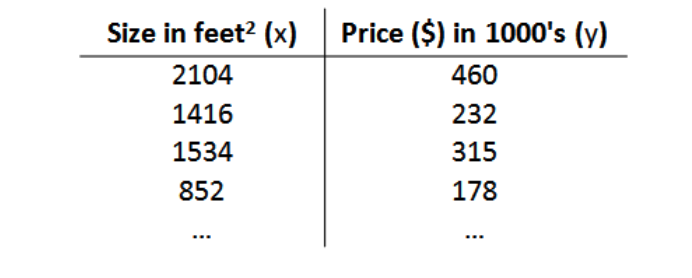• (m) ：代表训练集中实例的数量
• (x) ：代表特征/输入变量
• (y) ：代表目标变量/输出变量
• (left( x,y ight)) ：代表训练集中的实例
• (({{x}^{(i)}},{{y}^{(i)}}))： 代表第(i) 个观察实例
• (h) ：代表学习算法的解决方案或函数也称为假设（hypothesis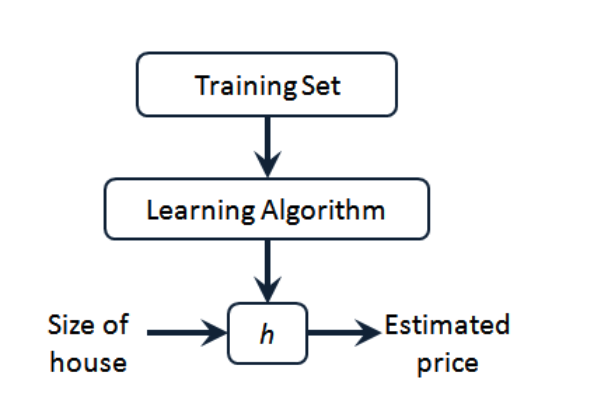## 2 代价函数

[h_ heta left( x ight)= heta_{0}+ heta_{1}x
]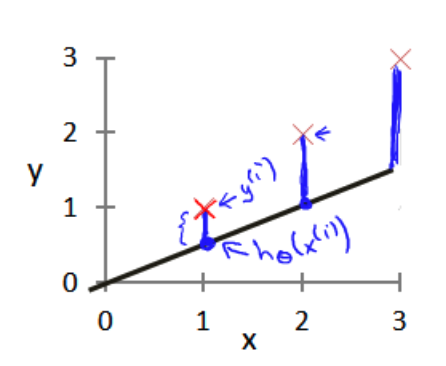[J left( heta_0, heta_1 ight) = frac{1}{2m}sumlimits_{i=1}^m left( h_{ heta}(x^{(i)})-y^{(i)} ight)^{2}
]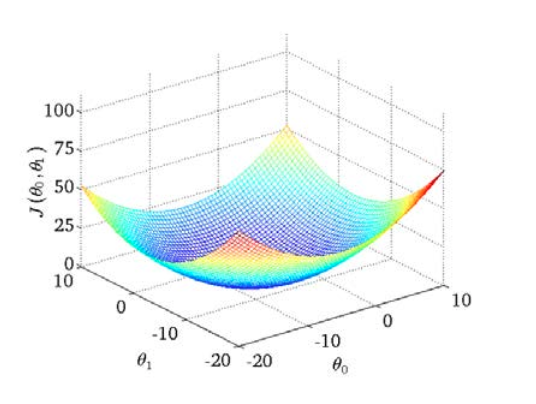[ underset{ heta_{0}, heta_{1}}{operatorname{minimize}}J( heta_{0}, heta_{1})
]

## 3 梯度下降

• Step 1 ：随机选择一个初始参数 (left( { heta_{0}},{ heta_{1}},……,{ heta_{n}} ight))，计算代价函数，

• Step 2 ：寻找下一个能让代价函数值下降最多的参数组合。我们持续这么做直到找到一个局部最小值（local minimum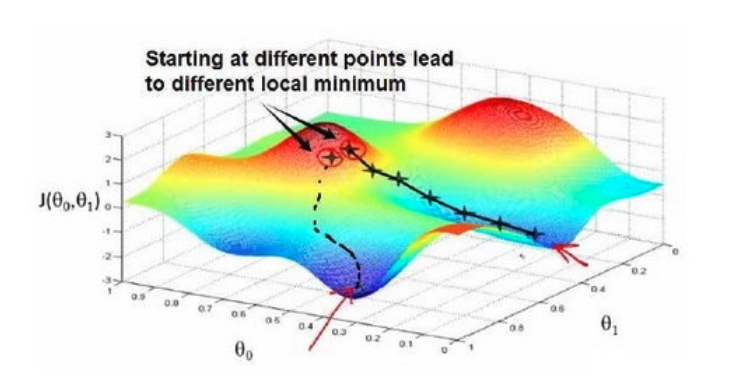• 一次更新多个参数
[egin{array}{l} ext { repeat until convergence { } \ left.qquad heta_{j}:= heta_{j}-alpha frac{partial}{partial heta_{j}} Jleft( heta_{0}, heta_{1}ight) quad ext { (for } j=0 ext { and } j=1ight) \ }end{array}
]

[alpha frac{partial }{partial {{ heta }_{0}}}J({{ heta }_{0}},{{ heta }_{1}})
\
alpha frac{partial }{partial {{ heta }_{1}}}J({{ heta }_{0}},{{ heta }_{1}})
]

[egin{array}{l} ext { temp } 0:= heta_{0}-alpha frac{partial}{partial heta_{0}} Jleft( heta_{0}, heta_{1}ight) \ ext { temp } 1:= heta_{1}-alpha frac{partial}{partial heta_{1}} Jleft( heta_{0}, heta_{1}ight) \ heta_{0}:= ext { temp } 0 \ heta_{1}:= ext { temp } 1end{array}
]

## 4 梯度下降的直观理解

[{ heta_{j}}:={ heta_{j}}-alpha frac{partial }{partial { heta_{j}}}Jleft( heta ight)
]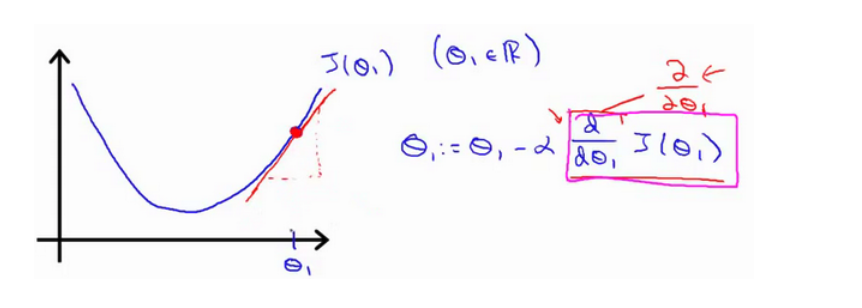[{ heta_{j}}:={ heta_{j}}-alpha frac{partial }{partial { heta_{j}}}Jleft( heta ight)
]

• (a) 太小了，学习速率太小，耗时大

• (a) 太大，可能会越过最低点，甚至可能无法收敛。

## 5 梯度下降的线性回归

[frac{partial }{partial {{ heta }_{j}}}J({{ heta }_{0}},{{ heta }_{1}})=frac{partial }{partial {{ heta }_{j}}}frac{1}{2m}{{sumlimits_{i=1}^{m}{left( {{h}_{ heta }}({{x}^{(i)}})-{{y}^{(i)}} ight)}}^{2}}
]

(j=0) 时：

[frac{partial }{partial {{ heta }_{0}}}J({{ heta }_{0}},{{ heta }_{1}})=frac{1}{m}{{sumlimits_{i=1}^{m}{left( {{h}_{ heta }}({{x}^{(i)}})-{{y}^{(i)}} ight)}}}
]

(j=1) 时：

[frac{partial }{partial {{ heta }_{1}}}J({{ heta }_{0}},{{ heta }_{1}})=frac{1}{m}sumlimits_{i=1}^{m}{left( left( {{h}_{ heta }}({{x}^{(i)}})-{{y}^{(i)}} ight)cdot {{x}^{(i)}} ight)}
]

[egin{array}{l} ext { Repeat { } \ qquad egin{array}{l} heta_{0}:= heta_{0}-a frac{1}{m} sum_{i=1}^{m}left(h_{ heta}left(x^{(i)}ight)-y^{(i)}ight) \ heta_{1}:= heta_{1}-a frac{1}{m} sum_{i=1}^{m}left(left(h_{ heta}left(x^{(i)}ight)-y^{(i)}ight) cdot x^{(i)}ight)end{array} \ }end{array}
]

## 6 多变量情形

### 6.1 形式变化

[h_{ heta}left( x ight)={ heta_{0}}+{ heta_{1}}{x_{1}}+{ heta_{2}}{x_{2}}+…+{ heta_{n}}{x_{n}}
]

[h_{ heta} left( x ight)={ heta_{0}}{x_{0}}+{ heta_{1}}{x_{1}}+{ heta_{2}}{x_{2}}+…+{ heta_{n}}{x_{n}}
]

[egin{array}{l} ext { Repeat }{ \ qquad heta_{mathrm{j}}:= heta_{mathrm{j}}-alpha frac{1}{mathrm{~m}} sum_{mathrm{i}=1}^{mathrm{m}}left(left(mathrm{h}_{ heta}left(mathrm{x}^{(mathrm{i})}ight)-mathrm{y}^{(mathrm{i})}ight) cdot mathrm{x}_{mathrm{j}}^{(mathrm{i})}ight)
\
~~~~~~~~~ ext { ( simultaneously update } heta_{mathrm{j}} ext { for } mathrm{j}=0,1, ldots, mathrm{n}) \ quad}end{array}
]

### 6.2 特征缩放

• 尺寸为 (0sim 2000)
• 房间数量为 (0sim 5)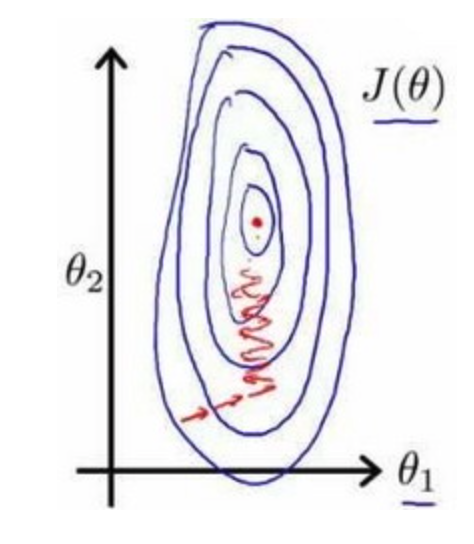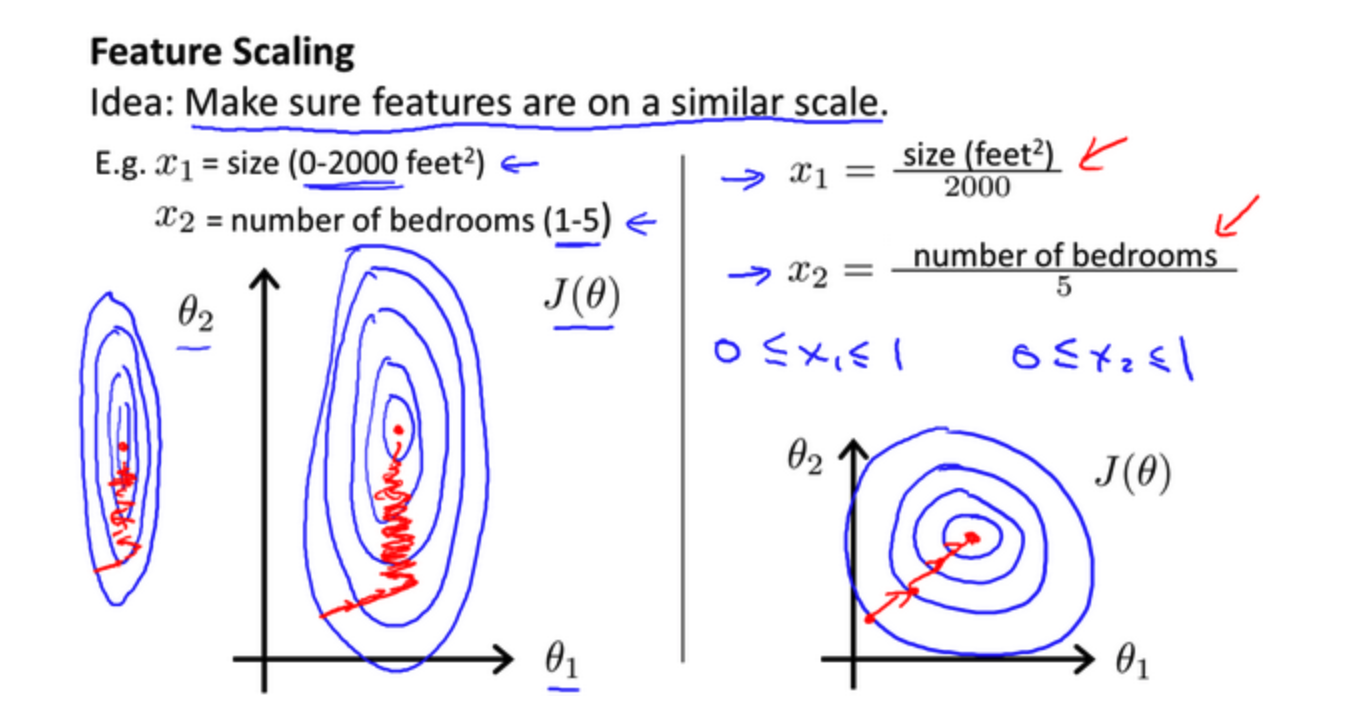[x = frac{x – mu}{s}
]

## 7 正则化

• 过拟合问题一般由于计算的多项式中的高次项特征造成，为了避免这些高次项特征的系数过大，一般的处理方法主要有以下几种
• 使用特征选择工具例如 `PCA` 选取影响大的特征
• 正则化，保留所有的特征，通过加入惩罚项减少部分高次项特征的系数

[{h_ heta}left( x ight)={ heta_{0}}+{ heta_{1}}{x_{1}}+{ heta_{2}}{x_{2}^2}+{ heta_{3}}{x_{3}^3}+{ heta_{4}}{x_{4}^4}
]

• 参数 ( heta_3、 heta_4) 是高次项的系数，目的是减少这两个参数的值

[underset{ heta }{mathop{min }}\,frac{1}{2m}[sumlimits_{i=1}^{m}{{{left( {{h}_{ heta }}left( {{x}^{(i)}} ight)-{{y}^{(i)}} ight)}^{2}}]}
]

[underset{ heta }{mathop{min }}\,frac{1}{2m}[sumlimits_{i=1}^{m}{{{left( {{h}_{ heta }}left( {{x}^{(i)}} ight)-{{y}^{(i)}} ight)}^{2}}+1000 heta _{3}^{2}+10000 heta _{4}^{2}]}
]

[Jleft( heta ight)=frac{1}{2m}[sumlimits_{i=1}^{m}{{{({h_ heta}({{x}^{(i)}})-{{y}^{(i)}})}^{2}}+lambda sumlimits_{j=1}^{n}{ heta_{j}^{2}}]}
]

• (lambda) 称作是正则化参数，也是待优化的一个参数
• 如果 (lambda) 过大，所有参数都会变小最终导致 (h_ heta(x) = heta_0)，导致欠拟合
• 如果 (lambda) 过小，不能很好的惩罚造成过拟合的特征项

[egin{align}
Repeat~~until~~conver&gence { ~~~~~~~~~~~~~~~~~~~~~~~~~~~~~~~~~~~~~~~~~~~~~~~~~~~~~~~~~~~~~~~~~~~~~~~~~~~~~~~~~~~~~~~~~~~~~~~~~~~~~~~~~~~~\
&{ heta_0}:={ heta_0}-afrac{1}{m}sumlimits_{i=1}^{m}{(({h_ heta}({{x}^{(i)}})-{{y}^{(i)}})x_{0}^{(i)}})
\
&{ heta_j}:={ heta_j}-a[frac{1}{m}sumlimits_{i=1}^{m}{(({h_ heta}({{x}^{(i)}})-{{y}^{(i)}})x_{j}^{left( i ight)}}+frac{lambda }{m}{ heta_j}]
\
&for j=1,2,…n \
}~~~~~~~~~&
end{align}
]

[{ heta_j}:={ heta_j}(1-afrac{lambda }{m})-afrac{1}{m}sumlimits_{i=1}^{m}{({h_ heta}({{x}^{(i)}})-{{y}^{(i)}})x_{j}^{left( i ight)}}
]